﻿ Optimal House Shape# Optimal House Shape

Disclaimer: This posting is just a light-weight excuse, in my last posting of this year, to perform a bit of calculus. I'm not seriously suggesting this is the technique engineers use to find the optimal pitches for rooves!

If I have a box that is a square based, topped with a square based pyramid (to make a, vaguely, house shaped volume), what are the optimal dimensions to minimize the surface area? (The premise here is that heat is transferred through surfaces, and we want to maximize the internal volume possible for living space using the smallest amount of material).

Here is a generic picture of such a construction. Let’s define the dimensions of the sides to be 2a, with height of b. We’ll define the height of the pyramid cap to be c.If we define the house has having unit volume, then we can derive an equation for the surface area and minimize this. Off we go …

### Equations

First let's calculate the volume. The volume of the house is the volume of the lower cuboid section plus the volume of the roof section (a square based pyramidal hat). We're defining this total volume as one, to represent unit volume.Next we'll work out the surface area. The surface area of the shape is the square base, plus four sides, plus the four triangles on the roof. (Yes, I'm including the floor in my calculation; let's imagine that I'm going to 3D print little Monopoly™ pieces and want to make them as volumous as possible with the least amount of material).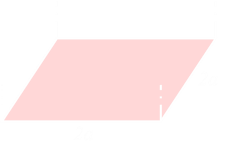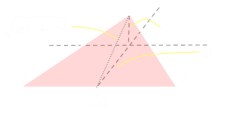Rearranging the equation for the volume gives an equation describing the height b.We can then substitute this into the equation for surface area.Differentiating, with respect to c and setting this to zero determines the turning point:Rearranging gives the value for c at the turning point.Putting this back into the equation for A, then differentiating this with respect to a and setting to zero for the turning point.With the value of a determined, we can substitute back to find the values for b and c.

### Results

Here are the results:

VarValue
2a1.0464
b0.7572
c0.4680

Here is a side rendering to scale: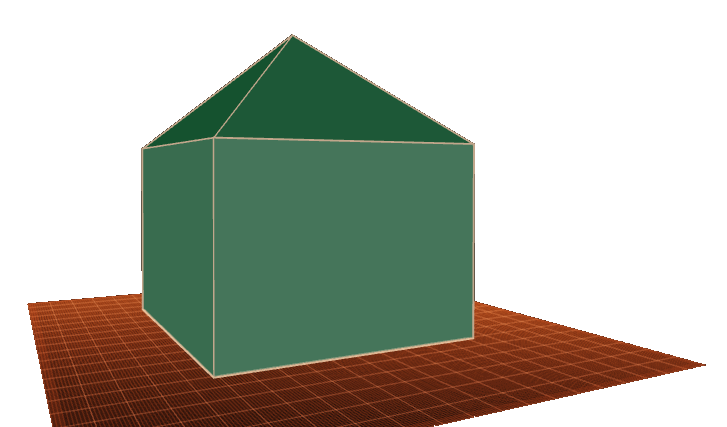Above is a 3D rendering. It looks vaguely house shaped.

## Comparisons to other solids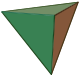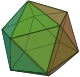The total surface area of the unit volume house shape is 5.733 units squared. How does this compare to other shapes?

A house shape is a more efficient shape than a cube. A unit volume cube has a surface area of 6 units squared.

At the extreme is a unit volume sphere, which has a surface area to volume ratio of 4.836 units squared. This is most efficient shape.

Here are the surface area to volume ratios for these and the unit volume platonic solids:

ShapeS/V ratio
Tetrahedron7.206Cube6.000Optimal House5.733Octahedron5.719Dodecahedron5.312Icosahedron5.148Sphere4.836You can find a complete list of all the articles here.Click here to receive email alerts on new articles.